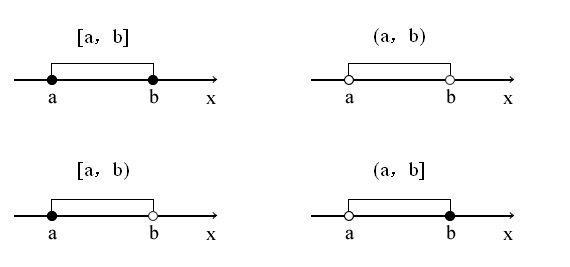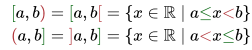# Closed Interval / Open Interval: Definition

Watch the video for an overview and examples of closed, open, half-closed and half-open intervals:

## 1. What is a Closed Interval?

In simple terms, a closed interval represents all possible numbers in a particular set. For example, the numbers 1, 2, 3, and 4 can be represented by the set {1, 2, 3, 4} or the closed interval [1, 4]. More formally, the definition of a closed interval is an interval that includes all of its limits.

## Notation

Intervals are designated by writing the start point and end point as an ordered pair, within brackets. Square brackets show that an endpoint is included in an interval; so the closed interval beginning at x and ending at y would be denoted [x, y].

For example, say you had a set of numbers {1, 2, 3, 4}. The closed interval could be written as [1, 4].

Intervals which begin or end at infinity can’t include the endpoint, so a standard parentheses is used; but since the limit is included in the interval they can still be closed. For example, closed intervals include:

• [x, ∞),
• (-∞ ,y],
• [∞, -∞].

## More Precise Definition

Ab interval is more precisely defined as a set of real numbers such that, for any two numbers a and b, any number c that lies between them is also included in the set.

• If the starting and ending point of the interval are finite numbers, these are included in the interval (“finite” just means bounded; it’s the opposite of infinite).
• If either the start or end point is infinite, the interval can’t be said to contain its endpoint (or start point) but if it does contain its limit point it can still be closed.

## 2. Open Intervals

Open intervals are defined as those which don’t include their endpoints. For example, let’s say you had a number x, which lies somewhere between zero and 100:

• The open interval would be (0, 100).
• The closed interval—which includes the endpoints— would be [0, 100].

Closed and opened intervals complement each other, but they aren’t mutually exclusive. The empty interval 0 and the interval containing all the reals, (∞, -∞), are actually both open and closed.

## 3. Half-Closed and Half-Open

An interval which includes one limit and not the other is half-closed; If it includes one endpoint and not the other it is half-open.

With closed interval, the endpoints are included in the interval. For an open interval, the endpoints are excluded. So if an interval is half-closed, one of the endpoints are included and the other isn’t.

## Half Closed Interval Notation

We denote an open interval by (a, b) and a closed interval by [a,b]; We denote a half-closed interval by a mixture of those two notations. Imagine your interval has endpoints a and b:

• If a is included and b isn’t, we can say the interval is [a,b).
• If b is included and a isn’t, we write it as (a,b].

It looks like mismatched brackets, but it’s really just a clever way of denoting which side is in the interval and which side isn’t.There’s one more way of denoting open intervals which you may not have come across yet: with backward square brackets. Using that notation, your half closed intervals become [a,b[ and ]a,b].

Here we use mathematical set notation to define these phrases:## Other Notation

Remember what an optimist calls a half-empty glass of water? Yes, half-full! The same principle works here, and an interval such as [a, b) can just as well be called half open. Your call.

For more on this topic, see: Interval Notation.

## 4. Interval Function

In simple terms, an interval function deals with inexact data—data that has to be represented with an interval. Interval functions are a part of interval analysis (a specific case of set-valued analysis), which handles uncertainty in intervals that commonly appear in real-world, deterministic phenomena (Chalco-Cano et al., 2013). For example, areas that often deal with inexact data include computer graphics, experimental physics, robotics, and many others (Budak et al. 2019). The most general type of differentiability for interval-valued functions is Hukuhara differentiability.

An early example of an interval function can be traced to Archimedes, who gave the following interval for π:The idea of interval-valued functions was however, largely forgotten until it’s resurrection in the latter half of the 20th century (Zhao, 2019).

## Example: A Class of Interval Function

One widely-used interval function class includes interval-valued objective functions in interval (fuzzy) linear or nonlinear programming. The class is defined as follows (Chalco-Cano et al., 2013):

F(t) = C · g(t),

Where:

## Software

Interval analysis is fairly esoteric, so you won’t find it widely implemented in software. Many programs have add-ons. For example, in Maple, interval functions can be implemented with the intpakX package (Kramer & Geulig, 2001). They include &sqr, &sqrt, &ln, &exp, &∗∗, &intpower (i.e. xn), &sin, &cos, &tan, &arcsin, &arccos, &arctan, &sinh, &cosh und &tanh.

## References

Budak, H. et al., (2019) Fractional Hermite-Hadamard-Type Inequalities for Interval-Valued Functions. Proceedings of the American Mathematical Society. Volume 148, Number 2, Feb. Pages 705-718.
Chalco-Cano, Y. et al., (2013). Calculus for interval-valued functions using generalized Hukuhara derivative and applications. Retrieved May 27, 2020 from: http://victorayala.cl/wp-content/uploads/41ref.pdf
Hunter, John K. Topology of the Real Numbers. From Introduction to Analysis. Retrieved from https://www.math.ucdavis.edu/~hunter/intro_analysis_pdf/ch5.pdf on June 23, 2018
LaValle, Steven. Topological Spaces: Closed Sets. Planning Algorithms, 2006, Cambridge University Press. Retrieved from http://planning.cs.uiuc.edu/node126.html on June 23, 2018.
Kramer, W. & Geulig, I. (2001). Interval Calculus in Maple. The Extension intpakX to the Package intpak of the Share-Library. Retrieved May 27, 2020 from:
http://www2.math.uni-wuppertal.de/org/WRST/preprints/prep_01_2.pdf
Oregon State University. Interval. Retrieved January 5, 2020 from: https://oregonstate.edu/instruct/mth251/cq/Glossary/gloss.interval.html
Ross, K. Elementary Analysis: The Theory of Calculus (Undergraduate Texts in Mathematics) 2nd ed. 2013 Edition. Springer.
Zhao, D. (2019). Chebyshev type inequalities for interval-valued functions. https://doi.org/10.1016/j.fss.2019.10.006

CITE THIS AS:
Stephanie Glen. "Closed Interval / Open Interval: Definition" From StatisticsHowTo.com: Elementary Statistics for the rest of us! https://www.statisticshowto.com/calculus-definitions/closed-interval-open-interval/